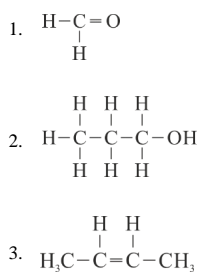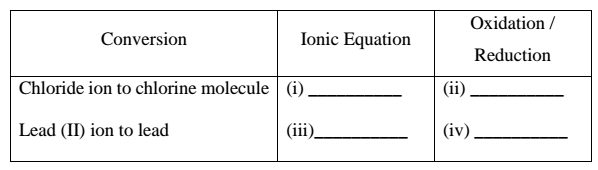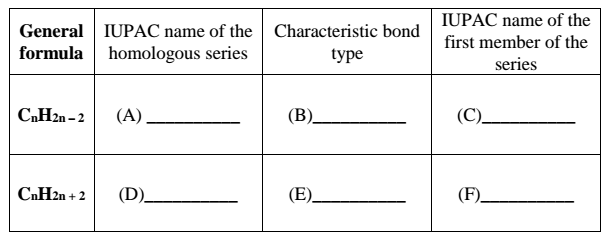# ICSE Class 10 Chemistry 2018 Question Paper

Section I is compulsory. You have to attempt any four questions from Section II.

### Section I (40 Marks)

Attempt all questions from this Section

Question 1

(a) Choose the correct answer from the options given below: 

(i) The salt solution which does not react with ammonium hydroxide is:
A. Calcium Nitrate
B. Zinc Nitrate
D. Copper Nitrate

(ii) The organic compound which undergoes substitution reaction is:
A. C2H2
B. C2H4
C. C10H18
D. C2H6

(iii) The electrolysis of acidified water is an example of:
A. Reduction
B. Oxidation
C. Redox reaction
D. Synthesis

(iv) The IUPAC name of dimethyl ether is:
A. Ethoxy methane
B. Methoxy methane
C. Methoxy ethane
D. Ethoxy ethane

(v) The catalyst used in the Contact Process is:
A. Copper
B. Iron
D. Manganese dioxide

(b) Give one word or a phrase for the following statements: 

(i) The energy released when an electron is added to a neutral gaseous isolated atom to form a negatively charged ion.

(ii) Process of formation of ions from molecules which are not in ionic state.

(iii) The tendency of an element to form chains of identical atoms.

(iv) The property by which certain hydrated salts, when left exposed to atmosphere, lose their water of crystallization and crumble into powder.

(v) The process by which sulphide ore is concentrated.

(c) Write a balanced chemical equation for each of the following: 

(i) Action of concentrated sulphuric acid on carbon.

(ii) Reaction of sodium hydroxide solution with iron (III) chloride solution.

(iii) Action of heat on aluminum hydroxide.

(iv) Reaction of zinc with potassium hydroxide solution.

(v) Action of dilute hydrochloric acid on magnesium sulphite.

(d) (i) Give the IUPAC name for each of the following:(ii) Write the structural formula of the two isomers of butane.

(e) State one relevant observation for each of the following: 

(i) Lead nitrate solution is treated with sodium hydroxide solution drop wise till it is in excess.

(ii) At the anode, when molten lead bromide is electrolyzed using graphite electrodes.

(iii) Lead nitrate solution is mixed with dilute hydrochloric acid and heated.

(iv) Anhydrous calcium chloride is exposed to air for some time.

(v) Barium chloride solution is slowly added to sodium sulphate solution.

(f) Give a reason for each of the following: 

(i) Ionic compounds have a high melting point.

(ii) Inert gases do not form ions.

(iii) Ionisation potential increases across a period, from left to right.

(iv) Alkali metals are good reducing agents.

(v) Conductivity of dilute hydrochloric acid is greater than that of acetic acid.

(g) Name the gas that is produced in each of the following cases: 

(i) Sulphur is oxidized by concentrated nitric acid.

(ii) Action of dilute hydrochloric acid on sodium sulphide.

(iii) Action of cold and dilute nitric acid on copper.

(iv) At the anode during the electrolysis of acidified water.

(v) Reaction of ethanol and sodium.

(h) Fill up the blanks with the correct choice given in brackets. 

(i) Ionic or electrovalent compounds do not conduct electricity in their _____________ state. (fused / solid)

(ii) Electrolysis of aqueous sodium chloride solution will form _____________ at the cathode. (hydrogen gas / sodium metal)

(iii) Dry hydrogen chloride gas can be collected by _____________ displacement of air. (downward / upward)

(iv) The most common ore of iron is _____________. (calamine / haematite)

(v) The salt prepared by the method of direct combination is _____________. (iron (II) chloride / iron (III) chloride)

### Section II (40 Marks)

Attempt any four questions from this Section

Question 2

(a) (i) What do you understand by a lone pair of electrons?
(ii) Draw the electron dot diagram of Hydronium ion. (H=1; O=8) 

(b) In Period 3 of the Periodic Table, element B is placed to the left of element A.
On the basis of this information, choose the correct word from the brackets to complete the following statements:
(i) The element B would have (lower / higher) metallic character than A.
(ii) The element A would probably have (lesser / higher) electron affinity than B.
(iii) The element A would have (greater / smaller) atomic size than B. 

(c) Copy and complete the following table which refers to the conversion of ions to neutral particles.Question 3

(a) (i) Write the balanced chemical equation to prepare ammonia gas in the laboratory by using an alkali.
(ii) State why concentrated sulphuric acid is not used for drying ammonia gas.
(iii) Why is ammonia gas not collected over water? 

(b) (i) Name the acid used for the preparation of hydrogen chloride gas in the laboratory. Why is this particular acid preferred to other acids?
(ii) Write the balanced chemical equation for the laboratory preparation of hydrogen chloride gas. 

(c) For the preparation of hydrochloric acid in the laboratory:
(i) Why is direct absorption of hydrogen chloride gas in water not feasible?
(ii) What arrangement is done to dissolve hydrogen chloride gas in water? 

(d) For the electro-refining of copper:
(i) What is the cathode made up of?
(ii) Write the reaction that takes place at the anode. 

Question 4

(a) The percentage composition of a gas is:
Nitrogen 82.35%, Hydrogen 17.64%.
Find the empirical formula of the gas. [N = 14, H = 1] 

(b) Aluminum carbide reacts with water according to the following equation:
Al4C3 + 12H2O → 4Al(OH)3 + 3CH4
(i) What mass of aluminum hydroxide is formed from 12 g of aluminum carbide?
(ii) What volume of methane at s.t.p. is obtained from 12 g of aluminum carbide?
[Relative molecular weight of Al4C3 = 144; Al(OH)3 = 78] 

(c) (i) If 150 cc of gas A contains X molecules, how many molecules of gas B will be present in 75 cc of B?
The gases A and B are under the same conditions of temperature and pressure.
(ii) Name the law on which the above problem is based. 

(d) Name the main component of the following alloys:
(i) Brass
(ii) Duralumin 

Question 5

(a) Complete the following table which relates to the homologous series of hydrocarbons.(b) (i) Name the most common ore of the metal aluminum from which the metal is extracted. Write the chemical formula of the ore.
(ii) Name the process by which impure ore of aluminum gets purified by using concentrated solution of an alkali.
(iii) Write the equation for the formation of aluminum at the cathode during the electrolysis of alumina. 

Question 6

(a) A compound X (having vinegar like smell) when treated with ethanol in the presence of the acid Z, gives a compound Y which has a fruity smell.
The reaction is:
C2H5OH + X  →𝑍→  Y + H2O

(i) Identify Y and Z.
(ii) Write the structural formula of X.
(iii) Name the above reaction. 

(b) Ethane burns in oxygen to form CO2 and H2O according to the equation:
2C2H6 + 7O2 → 4CO2 + 6H2O.
If 1250 cc of oxygen is burnt with 300 cc of ethane.
Calculate:
(i) the volume of CO2 formed.
(ii) the volume of unused O2. 

(c) Three solutions P, Q and R have pH value of 3.5, 5.2 and 12.2 respectively.
Which one of these is a:
(i) Weak acid?
(ii) Strong alkali? 

Question 7

(a) Give a chemical test to distinguish between the following pairs of chemicals:
(i) Lead nitrate solution and Zinc nitrate solution
(ii) Sodium chloride solution and Sodium nitrate solution 

(b) Write a balanced equation for the preparation of each of the following salts:
(i) Copper sulphate from Copper carbonate.
(ii) Zinc carbonate from Zinc sulphate. 

(c) (i) What is the type of salt formed when the reactants are heated at a suitable temperature for the preparation of Nitric acid?
(ii) State why for the preparation of Nitric acid, the complete apparatus is made up of glass. 

(d) Which property of sulphuric acid is shown by the reaction of concentrated sulphuric acid with:
(i) Ethanol?
(ii) Carbon?